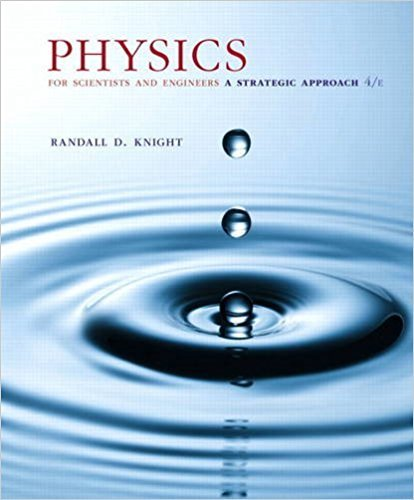×
×

# What are the rest energy, the kinetic energy, and theISBN: 9780134081496 191

## Solution for problem 36.74 Chapter 36

Physics for Scientists and Engineers: A Strategic Approach, Standard Edition (Chs 1-36) | 4th Edition

• Textbook Solutions
• 2901 Step-by-step solutions solved by professors and subject experts
• Get 24/7 help from StudySoup virtual teaching assistantsPhysics for Scientists and Engineers: A Strategic Approach, Standard Edition (Chs 1-36) | 4th Edition

4 5 1 416 Reviews
24
2
Problem 36.74

What are the rest energy, the kinetic energy, and the total energy of a 1.0 g particle with a speed of 0.80c?

Step-by-Step Solution:
Step 1 of 3

Week 5 Physics 2 for Engineering Chapter 24: Capacitance and Dielectrics Capacitors store charge C = Q/V Unit is Farads, 1 Farad = 1 C/V Review: Q = σ A V = Ed Parallel Plate Capacitor C = A Ɛ / 0 C = Q/V = σ A/ Ed = A Ɛ / d 0 Capacitors in series Charge on each capacitor is the same Voltage on each...

Step 2 of 3

Step 3 of 3

##### ISBN: 9780134081496

This full solution covers the following key subjects: . This expansive textbook survival guide covers 42 chapters, and 4463 solutions. Physics for Scientists and Engineers: A Strategic Approach, Standard Edition (Chs 1-36) was written by and is associated to the ISBN: 9780134081496. Since the solution to 36.74 from 36 chapter was answered, more than 243 students have viewed the full step-by-step answer. The answer to “What are the rest energy, the kinetic energy, and the total energy of a 1.0 g particle with a speed of 0.80c?” is broken down into a number of easy to follow steps, and 22 words. This textbook survival guide was created for the textbook: Physics for Scientists and Engineers: A Strategic Approach, Standard Edition (Chs 1-36), edition: 4. The full step-by-step solution to problem: 36.74 from chapter: 36 was answered by , our top Physics solution expert on 12/28/17, 08:06PM.

Unlock Textbook Solution